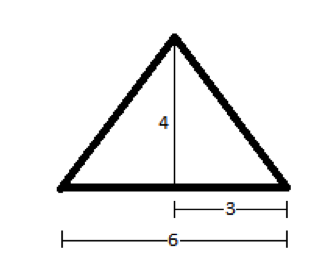# High School Math : How to find the length of the hypotenuse of an acute / obtuse triangle

## Example Questions

### Example Question #1 : How To Find The Length Of The Hypotenuse Of An Acute / Obtuse Triangle

An isosceles triangle has an area of 12. If the ratio of the base to the height is 3:2, what is the length of the two equal sides?

Possible Answers:

4√3

3√3

6

5

4

Correct answer:

5

Explanation:

Area of a triangle is ½ x base x height. Since base:height = 3:2, base = 1.5 height.  Area = 12 = ½ x 1.5 height x height or 24/1.5 = height2.  Height = 4.  Base = 1.5 height = 6. Half the base and the height form the legs of a right triangle, with an equal leg of the isosceles triangle as the hypotenuse. This is a 3-4-5 right triangle.### Example Question #2 : How To Find The Length Of The Hypotenuse Of An Acute / Obtuse Triangle

Two sides of a triangle each have length 6. All of the following could be the length of the third side EXCEPT

Possible Answers:
11
3
2
12
1
Correct answer: 12
Explanation:

This question is about the Triangle Inequality, which states that in a triangle with two sides A and B, the third side must be greater than the absolute value of the difference between A and B and smaller than the sum of A and B.

Applying the Triangle Inequality to this problem, we see that the third side must be greater than the absolute value of the difference between the other two sides, which is |6-6|=0, and smaller than the sum of the two other sides, which is 6+6=12. The only answer choice that does not satisfy this range of possible values is 12 since the third side must be LESS than 12.

### All High School Math Resources